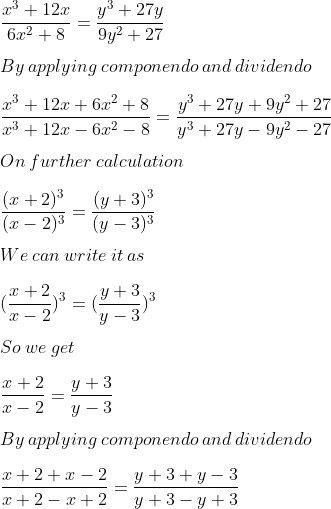Guru

# Give x³+12x/(6x²+8) =(y³+27y/9y²+27). Using componendo and dividendo find x : y.

• 0

exam oriented an important question from ML aggarwal, class 10th, chapter 7, ratio and proportion, Avichal publication

This question has been asked in previous year question paper 2015.

In this ques we have been given the equation and we have to find the value of x :y by using componendo and dividendo.

Question 17, 7.3

Share

1. Solution:

It is given thatBy further calculation

2x/4 = 2y/3

x/2 = y/3

By cross multiplication

x/y = 2/3

Hence, the required ratio x: y is 2: 3.

• 0